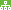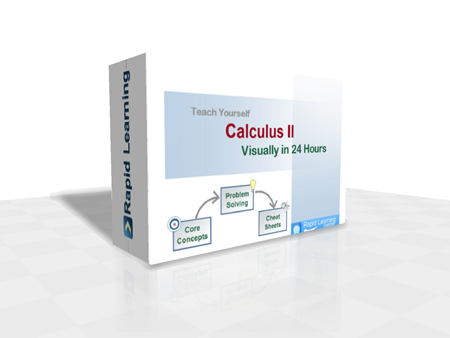How to Learn in 24 Hours?The Rapid Learning Movie

 Need Help? M-F: 9am-5pm(PST): Toll-Free: (877) RAPID-10 US Direct: (714) 692-2900 Int'l: 001-714-692-2900 24/7 Online Technical Support: The Rapid Support Center Secure Online Order:Need Proof? Testimonials by Our Users

 Rapid Learning Courses: Chemistry in 24 Hours Biology in 24 Hours Physics in 24 Hours Mathematics in 24 Hours Psychology in 24 Hours SAT in 24 Hours ACT in 24 Hours AP in 24 Hours CLEP in 24 Hours MCAT in 24 Hours (Medical) USMLE in 24 Hours (Boards) DAT in 24 Hours (Dental) OAT in 24 Hours (Optometry) PCAT in 24 Hours (Pharmacy) Nursing Entrance Exams Certification in 24 Hours eBook - Survival Kits Audiobooks (MP3)

 Tell-A-Friend: Have friends taking science and math courses too? Tell them about our rapid learning system.Home »  Mathematics » Calculus IICourse Preview

Calculus II Rapid Learning Series is the second of the three-course calculus sequence (I, II & III), designed typically for math, science and engineering majors or anyone who is required to take three semesters of calculus courses in college. This sequence of three courses cover the single variable and multivariable calculus. If you are required to take only one semester of calculus course, go for our College Calculus - RL411. If you are required to take three semesters of calculus courses, you should take our Calculus I, Calculus II and Calculus III, each represents one-semester of contents sequentially.

This Calculus II course includes foundation knowledge, calculus of transcendental functions, integration techniques, differential equations, parametric curves and polar coordinates, infinite sequences and series, with the focus on conceptual understanding and problem solving.

With the understanding of the fact that for many students calculus is a difficult subject, we adapt a more gentle and visual approach to calculus concepts. This ensures the learning of calculus is easy and fun, by watching the YouTube like videos and playing interactive drills.

Starting with your basic understanding of functions and graphs, we introduce the concept of limits visually and build the foundation of calculus. For the core units of derivative and integral, we illustrate each concept graphically and demonstrate how to solve each type of calculus problems step by step. We then help to build your critical skills in problem solving, graphing calculators and calculus exam with visualization and examples.

1. 24 Core Concept Tutorials (Flash Movies)
24x visual tutorials for 24 chapters, with concept map, concept illustration, step-wise problem solving and topical summary, narrated by college calculus professors.
2. 24 Problem-Solving Drills (Flash Games)
24x feedback based Quizzes, concept-based problems, summary reviews on all problems and scoring system to track performance.
3. 24 Super Review Cheat Sheets (PDF Printables)
One cheat sheet per topic, key concepts in a single-sheet, at-a-glance review of each topic and both printable and laminatible Ideal for exam prep quick review.
Pre-requisites for Calculus: (1) Elementary Algebra - RL409 (2) Intermediate Algebra - RL407 (3) Pre-Calculus - RL403.

Calculus II Rapid Learning Series

This 24-chapter series provides comprehensive coverage of traditional college calculus topics for any students who are taking multiple semester calculus courses.

Core Unit #1 – The Foundation

• Tutorial 01: Introduction to Calculus II
• Tutorial 02: Precalculus Review
• Tutorial 03: Review of Calculus I

Core Unit #2 – Calculus of Transcendental Functions

• Tutorial 04: Calculus of Exponential Functions
• Tutorial 05: Inverse Functions and Calculus of Logarithmic Functions
• Tutorial 06: Calculus of Inverse Trigonometric Functions, Hyperbolic Functions and Inverse Hyperbolic Functions

Core Unit #3 – Integration Techniques

• Tutorial 07: Basic Integration Rules, Integration by Parts, and Partial Fractions
• Tutorial 08: Trigonometric Integrals and Trigonometric Substitution
• Tutorial 09: Indeterminate Forms, L'Hopital's Rule and Improper Integrals

Core Unit #4 – Differential Equations

• Tutorial 10: Differential Equations: Analytical Methods
• Tutorial 11: Differential Equations: A Graphical Method and Mathematical Modeling
• Tutorial 12: Differential Equations: More Mathematical Modeling and A Numerical Method

Core Unit #5 – Parametric Curves and Polar Coordinates

• Tutorial 13: Plane Curves and Parametric Equations
• Tutorial 14: Calculus for Parametric Equations
• Tutorial 15: Polar Coordinates and Calculus
• Tutorial 16: Arc Length and Surface Area in Polar Coordinates
• Tutorial 17: Conic Sections and Calculus

Core Unit #6 – Infinite Sequences and Series

• Tutorial 18: Sequences, Series and Convergence
• Tutorial 19: Integral Test, Estimates of Sums and Comparison Test
• Tutorial 20: Alternating Series, the Ratio and Root Tests
• Tutorial 21: Power Series, Taylor Series, Maclaurin Series, Taylor Polynomials and Approximation

Core Unit #7 – Critical Skills

• Tutorial 22: Problem Solving in Calculus
• Tutorial 23: Graphing Utilities in Calculus
• Tutorial 24: Calculus Exam Guide

Master Calculus the rich-media way, get started now:

Course Preview